### Plane and Circularly Polarized EM Waves

Plane and Circularly Polarized EM Waves

Electromagnetic waves are transverse in the sense that associated electric and magnetic field vectors are both perpendicular to the direction of wave propagation. The Poynting vector defined by

S = E x H (W/m^2),

indicates not only the magnitude of the energy flux density (energy flow rate per unit area per unit time, Watts/m^2) but also the direction of energy flow. For simple electromagnetic waves, the Poynting vector is in the same direction as the wave vector, k.

The first animation shows propagation of sinusoidal plane electromagnetic waves in the z direction. The electric field is assumed in the x direction, and the magnetic field in the y direction.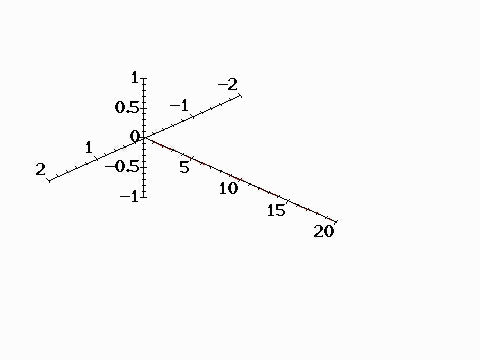A plane electromagnetic wave can be considered as vector combination of two circularly polarized waves rotating in opposite directions. The animation below shows propagation of electric field associated with a circularly polarized wave with postitive helicity. (Positive helicity is the case such that a screw would move in the direction of wave propagation if rotated with the electric field. In optics, it is called "left hand" circualr polarization. Negative helicity (right hand polarization) refers to rotation in the oppsite direction.) The moving end of the helix indicates the head of the electric field vector which is rotating about the z axis as shown in the right figure.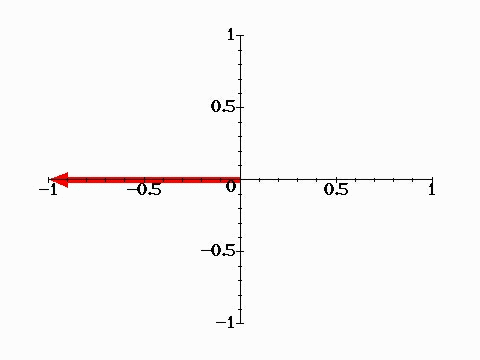Animation below shows vector sum of two circularly polarized waves with opposite helicities which results in formation of a plane wave. Electromagnetic waves emitted by charged particles undergoing circualr motion (e.g., electrons trapped in a magnetic field) are in general circularly (or elliptically) polarized. Circularly polarized waves carry angular momentum as well as energy and momentum. The angular mometum flux density is given by

R = r x (E x H)/c,

as discussed in Example 6, p. 161 of the textbook.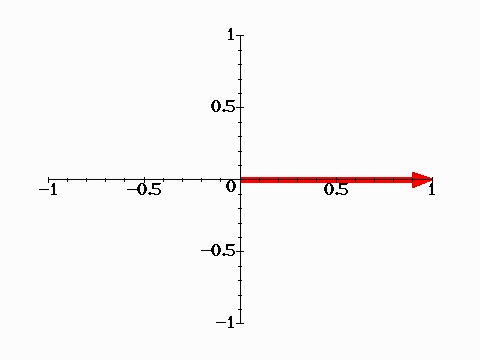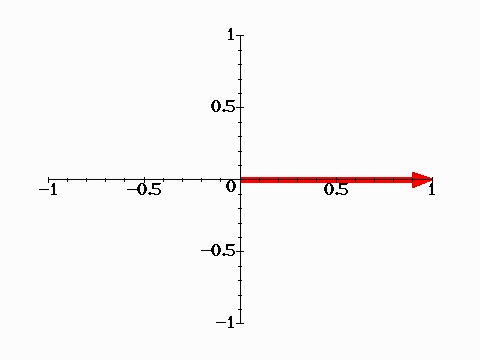图1 图2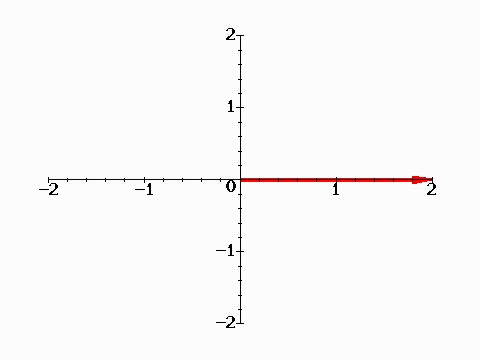To repeat animation, click "Back" then "Forward" buttons.
Ċ
Jason Lin,
2018年9月6日 凌晨12:43